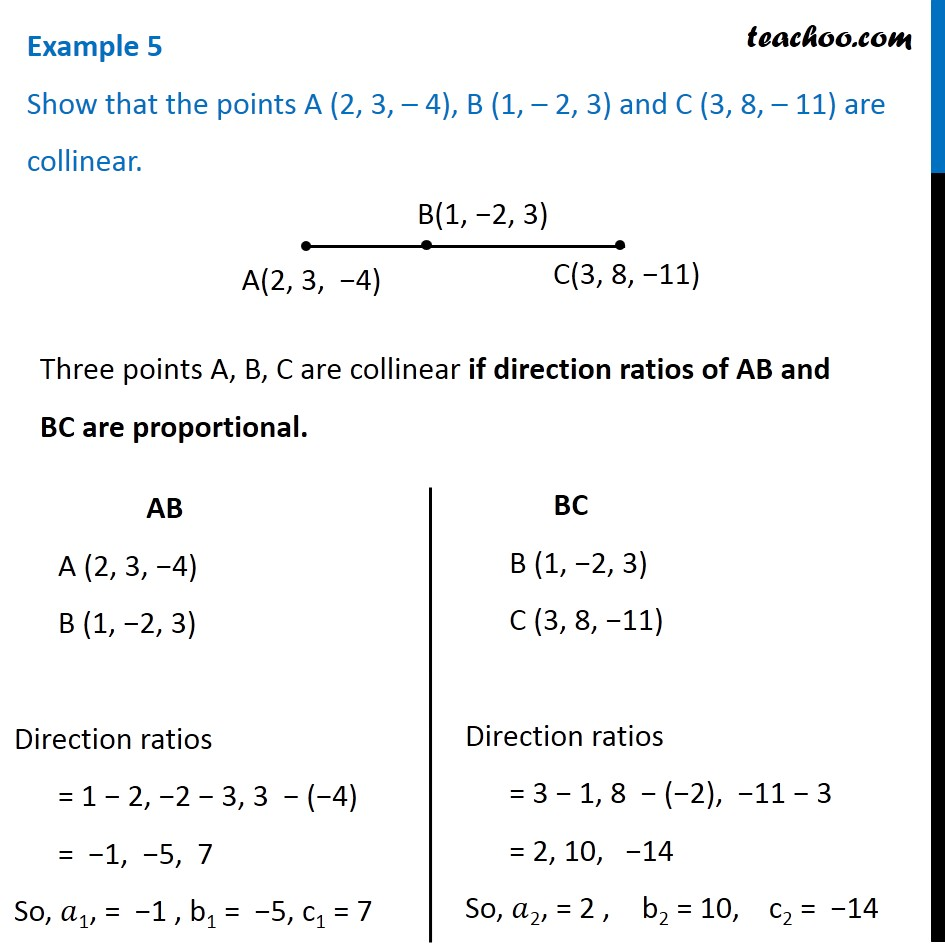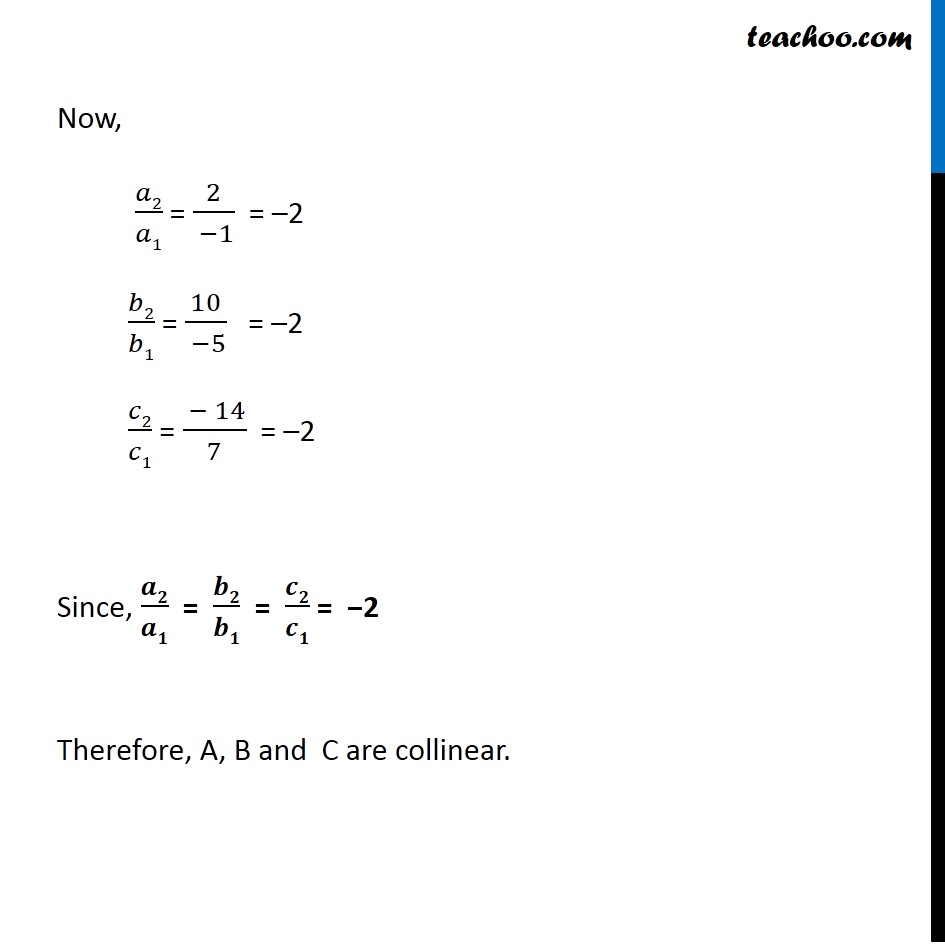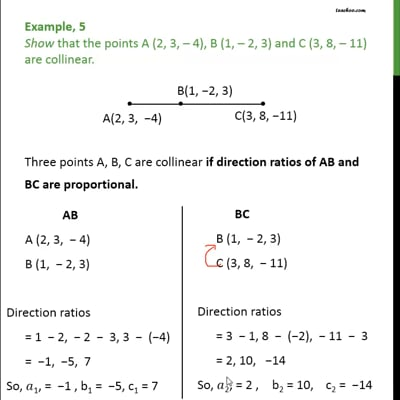Examples

Chapter 11 Class 12 Three Dimensional Geometry
Serial order wiseThis video is only available for Teachoo black users

Get live Maths 1-on-1 Classs - Class 6 to 12

### Transcript

Example 5 Show that the points A (2, 3, – 4), B (1, – 2, 3) and C (3, 8, – 11) are collinear. Three points A, B, C are collinear if direction ratios of AB and BC are proportional. AB A (2, 3, −4) B (1, −2, 3) Direction ratios = 1 − 2, −2 − 3, 3 − (−4) = −1, −5, 7 So, 𝑎1, = −1 , b1 = −5, c1 = 7 Now, 𝑎2/𝑎1 = 2/( −1) = –2 𝑏2/𝑏1 = 10/( −5) = –2 𝑐2/𝑐1 = ( − 14)/7 = –2 Since, 𝒂𝟐/𝒂𝟏 = 𝒃𝟐/𝒃𝟏 = 𝒄𝟐/𝒄𝟏 = −2 Therefore, A, B and C are collinear.